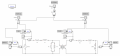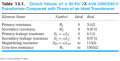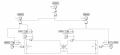#### jacky1997

Joined Oct 30, 2015
37
Good day everyone,

I'm trying to simulate a single phase transformator by using Simulink.
I've got the current values:
R1 = 3 Ohm
R2 = 0.03 Ohm
X1 = 6.5 Ohm
X2 = 0.07 Ohm
Rc = 100k Ohm
Xm = 15k Ohm
f = 60 Hz
Uprim = 2400V
Usec = 240V
S = 29kVA
cos Phi = 0.8

And I've made the current calculations:
L1 = X1/2*pi*f = 17,24 mH
L2 = X2/2*pi*f = 185.68 uH
Lm = Xm/2*pi*f = 39.79 H
S = Urms*Irms => Irms = 120.83 A
P = Urms*Irms*cos Phi = 23200 W
Q = Urms*Irms*sin Phi = 17400 VAr
Q>0 so Q = ohms-inductive => QL = 17400, QC = 0

I've put the following parameters in my transformator:
Nominal power(VA) and frequency: [29000 60]
Winding 1 parameters V1(rms), R1, L1: [2400 0 0], 0 because that is the ideal value and I've already put R1 and L1 in my circuit.
Winding 2 parameters V2(rms), R2, L2: [240 0 0], 0 because that is the ideal value and I've already put R2 and L2 in my circuit.
Magnetization resistance and inductance: [Inf Inf], Inf because that is the ideal value and I've already put Rc and Xm in my circuit.

I've put the following parameters in my LOAD:
Nominal voltage (rms): 240
Nominal frequency: 60
Active power (W): 23200
Inductive reactive power QL (positive VAr): 17400

I've made the current circuit and simulated it, but somehow my secondairy output voltage is only at 223.77 Vrms.
Can someone explain to me why that's the case? Did I do something wrong in my calculations or in my simulation?#### Papabravo

Joined Feb 24, 2006
18,778
Why do you think what you have drawn is an appropriate simulation of a transformer?
Why do you think that you get to specify the voltage at the load?

#### jacky1997

Joined Oct 30, 2015
37
Why do you think what you have drawn is an appropriate simulation of a transformer?
Why do you think that you get to specify the voltage at the load?
Well I don't know, that's why I'm here and that's the equivalent circuit of a transformer is it not? Honestly I don't really know what my output should be. I need to build a simulation of the following:It says 2400/240V so I assume my output has to be 240 Volts?

Last edited:

#### Papabravo

Joined Feb 24, 2006
18,778
I don't know what the simulator is doing but it looks like you are trying to model the behavior of the transformer with external components. It is hard to imagine how the simulator can untangle what is the behavior of what looks like external components and the transformer itself. If as you say those external components are modeling an ideal transformer then what is the actual transformer doing? I would start by eliminating the external components to see if I could get a simulation result that I understood and could explain.

#### jacky1997

Joined Oct 30, 2015
37
I don't know what the simulator is doing but it looks like you are trying to model the behavior of the transformer with external components. It is hard to imagine how the simulator can untangle what is the behavior of what looks like external components and the transformer itself. If as you say those external components are modeling an ideal transformer then what is the actual transformer doing? I would start by eliminating the external components to see if I could get a simulation result that I understood and could explain.
My apologies if it seemed confusing, but I've adjusted the simulation by removing the external parts and by entering the values in the transformator. The scope and math boxes are used to calculate the current, voltage, power and efficiency. My goal is to find out the voltage regulation and efficiency of this transformator, but I'm not sure if the transformator is 100% correct with the given values.#### Papabravo

Joined Feb 24, 2006
18,778
It seems like you get almost the same results with and without the external components. Is the transformer specified in terms of a turns ratio. If so, what is that ratio? Ideally it should be 10:1, so 2400 Vrms in should give 240 Vrms out. There are losses somewhere in the mix, but we cant see them.

#### jacky1997

Joined Oct 30, 2015
37
It seems like you get almost the same results with and without the external components. Is the transformer specified in terms of a turns ratio. If so, what is that ratio? Ideally it should be 10:1, so 2400 Vrms in should give 240 Vrms out. There are losses somewhere in the mix, but we cant see them.
Thanks for the reply, The losses are the Rc, Lm, R1, R2, L1 and L2. I've put the parameters into the transformer. Those are the core and copper losses. Ideally the output would be 240 Vrms, but because of the losses it won't reach the 240 Vrms. Is that what you mean? Now it pretty much makes sense to me thanks.

#### Papabravo

Joined Feb 24, 2006
18,778
My answer is a qualitative yes rather than a quantitative one. If you can do it, you could make one or all of those parameters ineffective to see if, in simulation at least, you can approach ideal behavior. Then I think you might have some confidence in the simulation results.